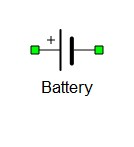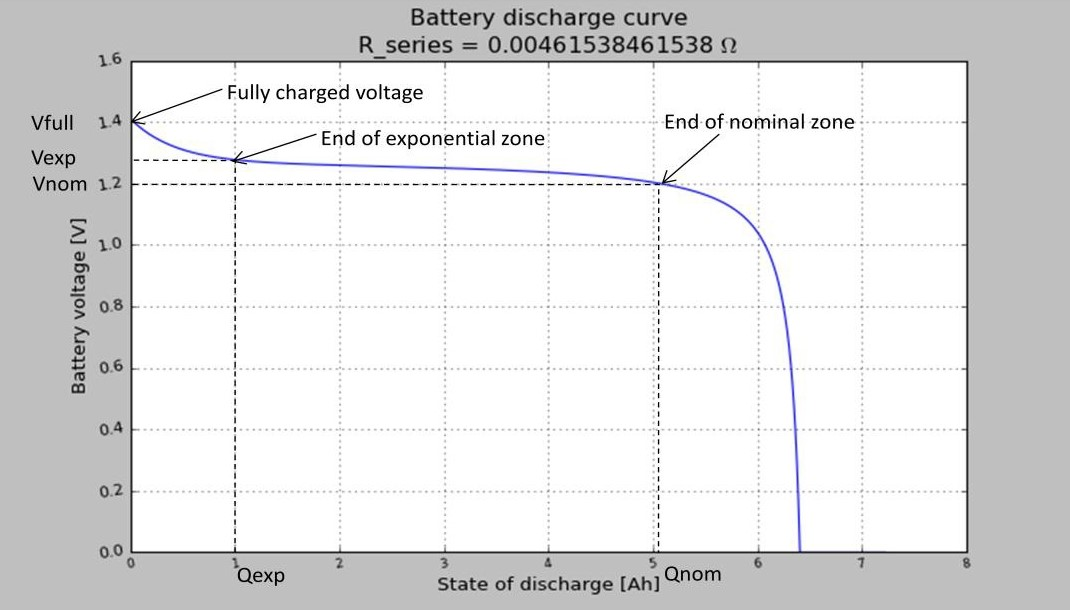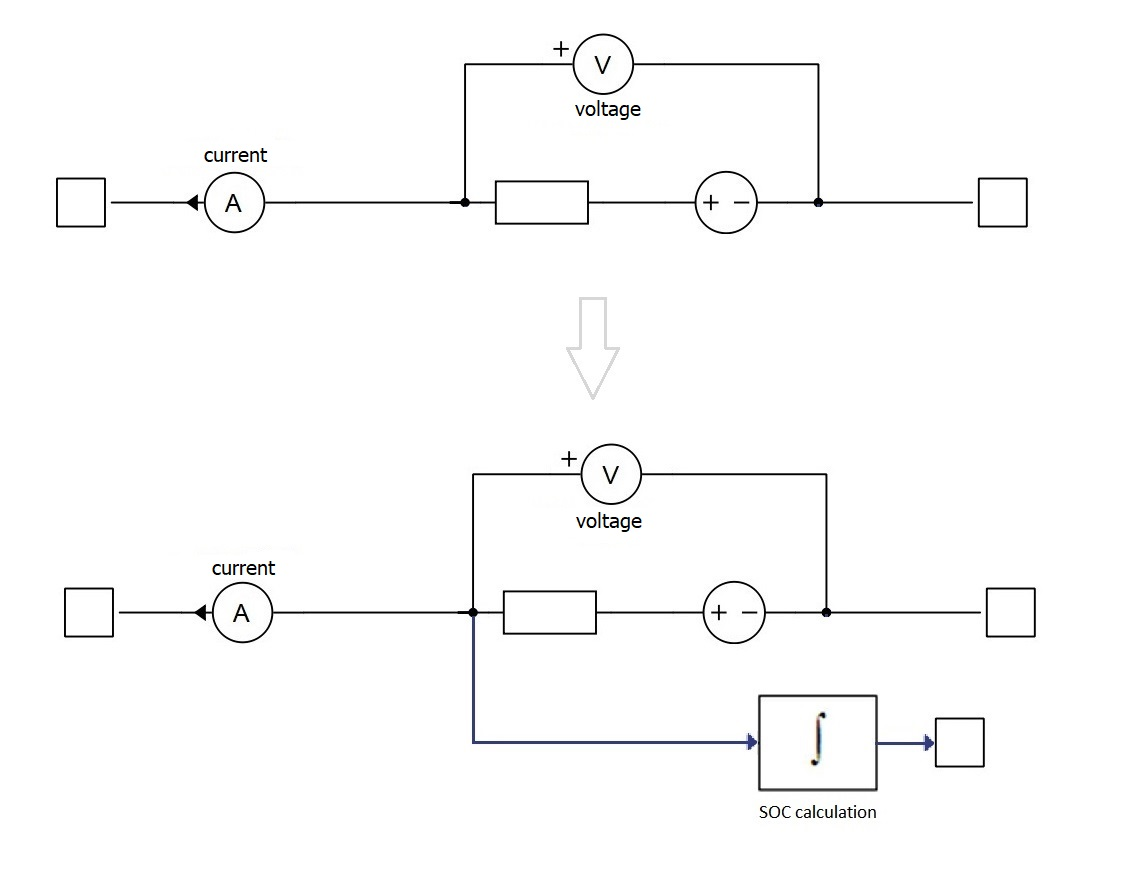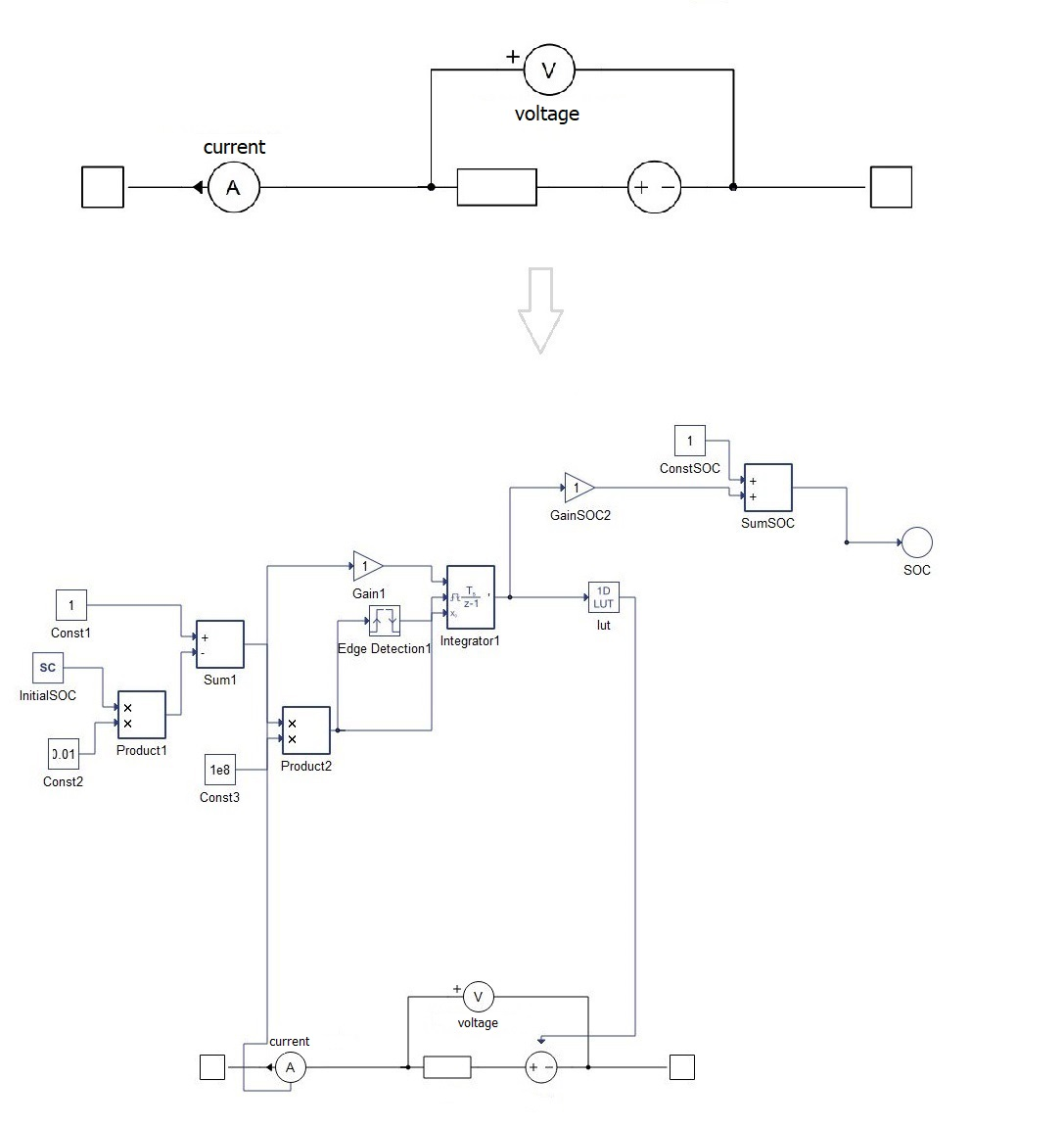# Battery

Description of the Battery component in Schematic Editor

The Battery component is implemented as a controlled voltage source and a series resistance. The voltage source is controlled by a lookup table that is automatically modified by changing the battery type. In order to get information regarding the battery's state of charge during simulation in HIL SCADA, it is necessary to check the State of charge output (SOC), and to connect the battery to the Probe, which adds the SOC signal (in %) to the list of analog signals in the SCADA.

Table 1. Battery component in Schematic Editor
component component dialog window component parametersBattery• General
• Battery type
• Nominal voltage (V)
• Capacity (Ah)
• Initial state of charge (%)
• Full charge voltage (%)
• Nominal discharge current (%)
• Internal resistance (ohm)
• Capacity at nominal voltage (%)
• Capacity at exponential zone (%)
• Voltage at exponential zone (%)
• Battery discharge curve preview button
• Signal processing
• State of charge output (SOC)
• Use signal processing Look up table
• Execution rate (s)

## Generic battery model

The battery model is implemented in the Typhoon emulator using a generic set of equations that describes the behavior of the battery.

There are 3 points at the battery's discharge curve (Figure 1) that are used by the equations:

1. Fully charged battery (Vfull, Q - rated capacity of the battery)
2. End of exponential zone (Vexp, Qexp)
3. End of nominal zone (Vnom, Qnom)Figure 1. Specific points marked on the battery discharge curve generated in Schematic Editor (example: Nickel-Metal-Hybride battery, 1.2V, 6.5Ah)

Figure 2 presents a schematic diagram of the battery model implemented in the Typhoon emulator. It consists of a controlled voltage source (E) and a series resistance (Rbat). The controlled voltage source is managed by the discharge state of the battery. The battery voltage is a function of the discharge state (it) according to the following formula:

$E={E}_{0}-K\frac{Q}{Q-it}+A*{e}^{-it*B}$

"it" in the previous equation is the discharge state of the battery, and it is defined as:

$it=\underset{0}{\overset{t}{\int }}ibat*dt$
• A: Voltage drop during the exponential zone: $A={E}_{full}-{E}_{exp}$
• 3/B: Charge at the end of exponential zone: $B=\frac{3}{{Q}_{exp}}$
• K: Polarization voltage: $K=\frac{\left({E}_{full}-{E}_{nom}+\mathrm{A}\mathrm{*}\left({\mathrm{e}}^{-\mathrm{B}\mathrm{*}{Q}_{nom}}-1\right)\right)\mathrm{*}\left(\mathrm{Q}-{Q}_{nom}\right)}{{Q}_{nom}}$
• Voltage constant E0: ${E}_{0}={E}_{full}+K+{R}_{bat}*i-A$

where i is the rated discharge current.

The series resistance is calculated by assuming the battery efficiency is 99.5%
${R}_{bat}={V}_{nom}\frac{1-0.995}{0.2*{Q}_{nom}}$

## Tab: General

All parameters for batteries are defined in Schematic Editor.

• Battery type: Models are available for the 4 most common battery types: Lead-Acid, Lithium-ion, Nickel-Cadmium and Nickel-Metal-Hydride. Additionally, you can choose to define your own battery composition.
• Nominal voltage: is the nominal voltage of the battery.
• Capacity: is the rated capacity of battery.
• Initial SOC: is the initial state of charge of the battery.

If you need additional parameters, then chose the "User defined" option. This enables the hidden parameters that allow you to define the battery's SOC curve in detail, letting you shape it to your needs.

• Full charge voltage: percentage of the nominal voltage of battery when it is fully charged. This number is always larger than 100%
• Nominal discharge current is defined as a percentage of the rated capacity/1h.
• Internal resistance
• Capacity at nominal voltage: percentage of the rated capacity.
• Capacity at exponential zone: percentage of the rated capacity.
• Voltage at exponential zone: percentage of the nominal voltage.
• Preview SOC Curve button will graph the characteristic SOC curve of a selected battery based on the previous equations (more documentation can be found in t-tn003 - Battery-modeling and application).

## Tab: Signal Processing

If the State of charge output (SOC) property is checked, an additional signal processing output port on the battery model will be added (Figure 6), which outputs the current state of charge of the battery. The Execution rate property will be enabled in that case, which determines the execution rate of the signal processing components.Figure 6. Schematic diagram of the battery model when State of charge output (SOC) property is checked

If the Use signal processing Look up table property is checked, the entire battery will be modeled with a Signal Processing lookup table (Figure 7). The initial State of Charge is set in HIL SCADA through the Scada Input property Name.InitialSOC. It can be changed during the simulation runtime. In this case, the Execution rate property will be enabled, adapting the simulation to the execution rate of the signal processing components and not by the time step defined in the circuit solver settings.Figure 7. Schematic diagram of the battery model when the Signal Processing Lookup Table property is checked

## Measurements internal to the battery component

Analog output variable name Description
name.Ia1 Battery output current
name Battery output voltage